# NCERT Solutions for Class 12 Physics Chapter 14 Semiconductor Electronics: Materials, Devices and Simple Circuits

In this chapter, we provide NCERT Solutions for Class 12 Physics Chapter 14 Semiconductor Electronics: Materials, Devices and Simple Circuits for English medium students, Which will very helpful for every student in their exams. Students can download the latest NCERT Solutions for Class 12 Physics Chapter 14 Semiconductor Electronics: Materials, Devices and Simple Circuits pdf, free NCERT solutions for Class 12 Physics Chapter 14 Semiconductor Electronics: Materials, Devices and Simple Circuits book pdf download. Now you will get step by step solution to each question. Class 12 physics is a very important subject for entrance exams like IIT JEE, CPMT, etc.

## NCERT Solutions for Class 12 Physics Chapter 14 Semiconductor Electronics Materials Devices And Simple Circuits

Question 1.
in an n-type silicon, which of the following statement is true?
(a) Electrons are majority carriers and trivalent atoms are the dopants.
(b) Electrons are minority carriers and pentavalent atoms are the dopants.
(c) Holes are minority carriers and pentavalent atoms are the dopants.
(d) Holes are majority carriers and trivalent atoms are the dopants.
Solution:
(c) For n-type silicon statement (c) is true.

Question 2.
Which of the statements given in previous question is true for p-type semiconductors?
Solution:
(d) For p-type semiconductors statement (d) is true.

Question 3.
Carbon, silicon and germanium have four valence electrons each.These are characterised by valence and conduction bands separated by energy band gap respectively equal to (Eg)c’ (Eg)si and (Eg)Ge. Which of the following statements is true?Solution:
(c) : The energy band gap is largest for carbon, less for silicon and least for germanium. So, the correct statement is (c).
(Eg)c > (Eg)si > (Eg)Ge

Question 4.
In an unbiased p-n junction, holes diffuse from the p-region to n-region because
(a) free electrons in the n-region attract them.
(b) they move across the junction by the potential difference.
(c) hole concentration in p-region is more as compared to n-region.
(d) all of the above.
Solution:
(c) : In the unbiased p-n junction, holes diffuse from the p-region to n-region because holes concentration in the p-region is high as compared to n-region.

Question 5.
When a forward bias is applied to a p-n junction, it
(a) raises the potential barrier.
(b) reduces the majority carrier current to zero.
(c) lowers the potential barrier.
(d) none of the above
Solution:
(c) : Under forward biasing the move¬ment of majority charge carriers across the junction reduces the width of depletion layer or lowers the potential barrier.

Question 6.
For transistor action, which of the following statements is/are correct:
(a) Base, emitter and collector regions should have similar size and doping concentrations.
(b) The base region must be very thin and lightly doped.
(c) The emitter junction is forward biased and collector junction is reverse biased.
(d) Both the emitter junction as well as the collector junction are forward biased.
Solution:
(b, c) For a transistor circuit in action, statements (b) and (c) are correct.

Question 7.
For a transistor amplifier, the voltage gain
(a) remains constant for all frequencies.
(b) is high at high and low frequencies and constant in the middle frequency range.
(c) is low at high and low frequencies and constant at mid frequencies.
(d) none of the above.
Solution:
(c) : The voltage gain in a transistor as amplifier is low at high and low frequencies and constant at mid frequencies.

Question 8.
In half-wave rectification, what is the output frequency if the input frequency is 50 Hz. What is the output frequency of a full-wave- rectifier for the same input frequency?
Solution:
In half wave rectification, only one ripple is obtained per cycle in the output.Output frequency of a half wave rectifier = input frequency = 50 Hz In full wave rectification, two ripples are obtained per cycle in the output.
Output frequency = 2 × input frequency = 2 × 50 = 100 Hz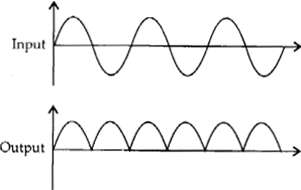Question 9.
For a CE-transistor amplifier, the audio signal voltage across the collector resistance of 2 kΩ is 2 V. Suppose the current amplification factor of the transistor is 100, find the input signal voltage and base current, if the base resistance is 1 kΩ
Solution:

Question 10.
Two amplifiers are connected one after the other in series (cascaded). The first amplifier has a voltage gain of 10 and the second has a voltage gain of 20. If the input signal is 0. 01 volt, calculate the output ac signal.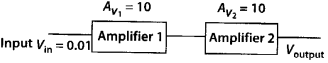Solution:
Total voltage gain can be calculated as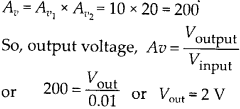Question 11.
A p-n photo diode is fabricated from a semiconductor with band gap of 2.8 eV. Can it detect a wavelength of 6000 nm?
Solution:
Energy of the incident photon with a band gap of 6000 nm.The photo diode need an energy of 2.8 eV to give response to incident light. As E < Eg, the given photo diode cannot detect the radiation of wavelength 6000 nm.
Question 12.
The number of silicon atoms per m3 is 5 × 1028. This is doped simultaneously with 5 × 1022 atoms per m3 of Arsenic and 5 × 1020 per m3 atoms of Indium. Calculate the number of electrons and holes. Given that n, = 1.5 × 1016 m3. Is the material n-type or p-type?
Solution:
We know that for each atom doped of Arsenic, one free electron is received. Similarly, for each atom doped of indium, a vacancy is created. So, the number of free electrons introduced by pentavalent impurity added ne = NAs = 5 × 1022 m-3
The number of holes introduced by trivalent impurity added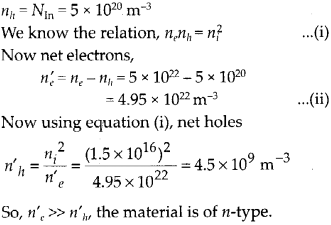Question 13.
In an intrinsic semiconductor the energy gap Eg is 1.2 eV. Its hole mobility is much smaller than electron mobility and independent of temperature. What is the ratio between conductivity at 600 K and that at 300 K? Assume that the temperature dependence of intrinsic carrier concentration n, is given bySolution:
Conductivity is given by σ = e (ne μe +nh μh) For intrinsic semiconductor ne = nh = ni Also mobility of holes (p,,) « mobility of electrons (pj So, conductivity a = enepe „ Temperature dependence of intrinsic carrier concentration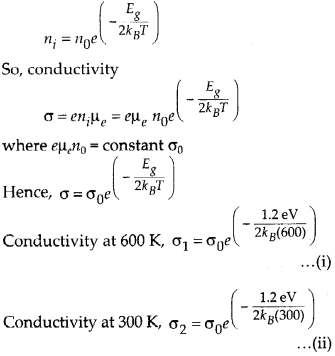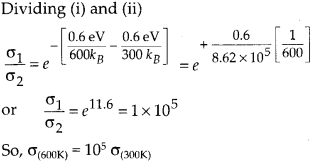Conductivity increases rapidly with the rise of temperature.

Question 14.
In a p-n junction diode, the current I can be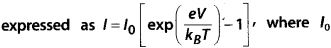is called the reverse saturation current, V is the voltage across the diode and is positive for forward bias and negative for reverse bias, and I is the current through the diode, kB is the Boltzmann constant (8.6 × 10-5 eV/K) and T is the absolute temperature. If for a given diode I0 = 5 × 10-12 A and T= 300 K, then
(a) What will be the forward current at a forward voltage of 0.6 V?
(b) What will be the increase in the current if the voltage across the diode is increased to 0.7 V?
(c) What is the dynamic resistance?
(d) What will be the current if reverse bias voltage changes from 1 V to 2 V?
Solution:
The current I through a junction diode is given as(a) When V = 0.6 V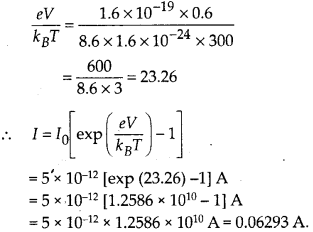(b) When V = 0.7 V,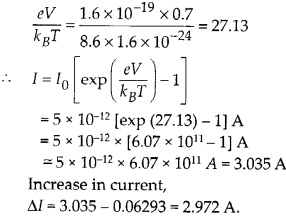(c)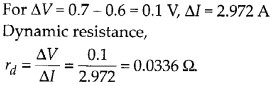(d) For both the voltages, the current I will be almost equal to I0, showing almost infinite dynamic resistance in the reverse bias. I=-I0= – 5 × 10-12 A.

Question 15.
YOU are given the two circuits as shown in figure. Show that circuit (a) acts as OR gate while the circuit (b) acts as AND gate.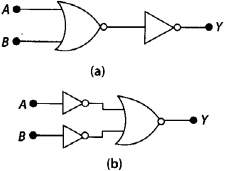Solution:
Let us first find the Boolean expression for logic circuit (a)By De-Morgan’s theorem we know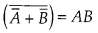so, above logic circuit provides output as AND gate

Question 16.
Write the truth table for a NAND gate connected as given in following figure.Hence identify the exact logic operation carried out by this circuit.
Solution:
The Boolean expression for NAND gate will beQuestion 17.
You are given two circuits as shown in figure which consist of NAND gates. Identify the logic operation carried out by the two circuits.Solution:
(a) Let us find Boolean expression for given logic circuit(b) By De-Morgan’s theoremso, the given logic circuit acts as OR gate.

Question 18.
Write the truth table for circuit given in figure below consisting of NOR gates and identify the logic operation (OR, AND, NOT) which this circuit is performing.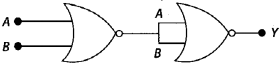(Hint: A – 0, B = 1 then A and B inputs of second NOR gate will be 0 and hence Y = 1. Similarly work out the values of Yfor other combinations of A and B. Compare with the truth table of OR, AND, NOT gates and find the correct one.)
Solution:
Let us find the Boolean expression for the logic circuit.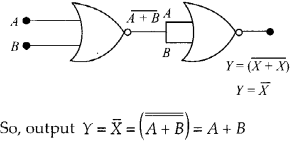Hence, the output of given logic circuit shows that logic circuit act as OR gate. Truth TableQuestion 19.
Write the truth table for the circuits given in figure consisting of NOR gates only. Identify the logic operations (OR, AND, NOT) performed by the two circuits.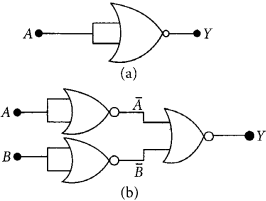Solution:
Boolean expression for logic circuit (a)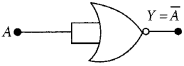Here the given NOR gate with short circuit input is acting as NOT gate. Its truth table isBoolean expression for logic circuit (b)Hence, the logic circuit acts like AND gate. Its truth table is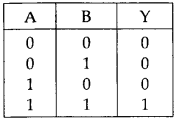All Chapter NCERT Solutions For Class12 Physics

—————————————————————————–

All Subject NCERT Solutions For Class12

*************************************************

Remark:

I think you got complete solutions for this chapter. If You have any queries regarding this chapter, please comment on the below section our subject teacher will answer you. We tried our best to give complete solutions so you got good marks in your exam.

If these solutions have helped you, you can also share Careerkundali.in to your friends.

Best of Luck!!